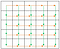# Kth Smallest Element in Sorted Matrix

In this post, I would like to share my thinking process for this problem.

Problem:

Given a n x n matrix where each of the rows and columns are sorted in ascending order, find the kth smallest element in the matrix.

Example:

`matrix = [   [ 1,  5,  9],   [10, 11, 13],   [12, 13, 15]],k = 8,return 13.`

Source : Leetcode 378

Let’s take some examples to understand better the problem.

`# example 1 : any number from row i+1 is higher than anyone from row i. But we can't establish any order between columns1 2 34 5 67 8 9# example 2: any number from col i+1 is higher than anyone from col i. But we can't establish any order between rows1 4 72 5 83 6 9# example 3: We can't establish any order between columns or rows1 2 53 4 8 6 7 9`

1st approach:

• Store the matrix in an array →O(N²)
• Sort the array → O(N²log(N²))
• Take the K th element → O(1)
=>
O(N²log(N²)) time complexity

Notice
In this approach:
1) we haven’t used the first property of the problem : rows are sorted
2) we haven’t used the second property of the problem : columns are sorted

So this solution is far from being the appropriate one !

2nd approach:

Instead of storing the matrix in an array and sorting it, let’s try to use at least the information that the rows are sorted.

We know that if we have 2 sorted arrays, we can merge them in O(N) time complexity.

So let’s do a linear merge. It consists of merging the first row with the second, then merge this result with the third row, then merge this result with the forth etc…

Merging 2 arrays does N+M comparisons in the worst case where N and M are the sizes of the arrays. So the time complexity of this solution is:

O(2N + 3N + 4N + … + (N-1)N) = O(N³)

Ok.. That makes it worse !

In fact, we can easily optimize this by trying a divide and conquer approach. It will merge the first row with the second, the third with the forth,… In the next level it will merge the result of merging the first 2 rows with the result of merging the 3rd and 4th row… And continue like this by levels until having all elements in the same array.

Time complexity : Each level takes O(N²) time complexity, and there are O(logN) levels, so the final time complexity is O(N²logN). Hmm.. We haven’t improved the first approach !

Take a step back

Let’s take a step back and draw our problem. This is usually a good way to think about solutions when we are stuck, but does not apply to all problems unfortunately.

Let’s draw arrows representing the order in the matrixArrows of order. In orange order within a row, in green order within a column

Interesting..Looks like an oriented graph . Let’s rotate the image 45 degrees to the right, and take some cells.

Indeed, these arrows make a graph, in which each element is smaller than its children.

The 1st smallest element is matrix. The second one can be either matrix or matrix.

If matrix is the second, then matrix and the children of matrix are the candidates of being the third smallest. The children of matrix are matrix and matrix.

Wait.. matrix is a child of matrix, it can’t be smaller ! So the only candidates are actually matrix and matrix.

So, what we need is to have a Data Structure that will help us retrieve the minimum element between the current candidates, remove that element and then insert its children in a reasonable time complexity.

We can go further and decide not to insert a child when there is still an ancestor of it in the DS (like matrix in the example above), but anyway let’s just insert the two children without any check, this will be sufficient.

And the most suitable data structure for retrieving minimums is … a priority queue !

Let’s see if we are good in terms of time complexity.

In the first iteration, the priority queue will store matrix (the only candidate for being the 1st smallest)

At each iteration we remove one element and insert at most 2 elements so the size of the priority queue will grow by 1 at most at each iteration.

At the kth iteration we will get the kth smallest element and there will be at most k elements in the priority queue.

Min deletion and Insertion in a priority queue takes O(log(Size))

So the time complexity is O(log(1)+log(2)+…log(K)) = O(Klog(K)). Which is good.

Here is the python implementation :

`def kthSmallest(self, matrix: List[List[int]], k: int) -> int:   if not matrix or k < 1: return   s = set()   s.add((0, 0))   heap = [(matrix, 0, 0)]   while k > 1:     top = heapq.heappop(heap)     row, col = top, top     if col+1 < len(matrix) and (row, col+1) not in s:        heapq.heappush(heap, (matrix[row][col+1], row, col+1))        s.add((row, col+1))     if row+1 < len(matrix) and (row+1, col) not in s:        heapq.heappush(heap, (matrix[row+1][col], row+1, col))        s.add((row+1, col))     k -= 1   return heap`

When we insert a value in the heap, we insert its position so that we know its children at removal. The children of matrix[row][col] are matrix[row+1][col] and matrix[row][col+1] (see the graph if you have a doubt).

We also need a set so that we don’t insert an element more than once in the heap.

Hope it helped ;)

Computer Science, Physics & Maths Enthusiast

## More from Ayoub Omari

Computer Science, Physics & Maths Enthusiast